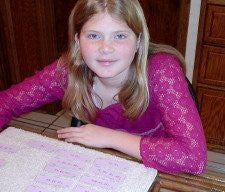• 46500

• Fourth grade materials for reinforcement
• Assorted formats. Most include answer sheets; cut materials include organization labels.
• Numerous exercise sets
Roman Numerals, Problem Solving D, Mixed Practice D, Fraction Concepts, Decimal Introduction, Rename, Estimation, Advanced Numeration, Fraction Number Lines, Sets (Cumulative), Order Fractions, Fraction Operations, More Fraction Operations, Inequalities, Number Theory, Decimal Line & Labels, Order Decimals, Problem Solving E, Mixed Practice E, More Advanced Numeration, Decimal Fraction Equivalence, Percent, Geometry Intermediate, Advanced Decimals, Advanced Geometry, Intervals (Whole Numbers), Intervals (Positive Reals), Intervals (Integers), Intervals (All Reals).